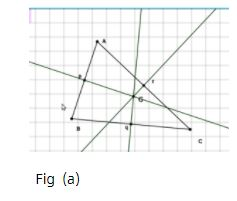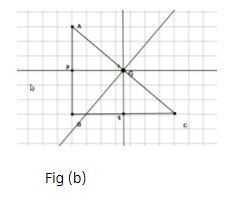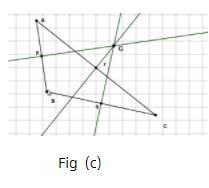# Procedure - To illustrate that Perpendicular Bisectors of the Sides of a Triangle Concur at a Point Class 9 Notes | EduRev

## Class 9 : Procedure - To illustrate that Perpendicular Bisectors of the Sides of a Triangle Concur at a Point Class 9 Notes | EduRev

The document Procedure - To illustrate that Perpendicular Bisectors of the Sides of a Triangle Concur at a Point Class 9 Notes | EduRev is a part of the Class 9 Course Mathematics (Maths) Class 9.
All you need of Class 9 at this link: Class 9

As performed in the real lab

Material Required:

Coloured paper, pencil, a pair of scissors, gum.

Procedure:

1. Cut an acute angled triangle from a coloured paper and name it as ABC.

2. Form the perpendicular bisector EF of AB using paper-folding method.

3. Similarly get the perpendicular bisectors GH and IJ of the sides AC and BC respectively.

4. Repeat the activity for right and obtuse angled triangles.

As performed in the simulator

1. Select three points A, B and C anywhere on the workbench  to draw a triangle.

2. Depending on your points selection acute, obtuse or right angled triangle is drawn.

3. Now, click on each mid-point to draw their respective perpendicular bisectors. You can use the scale to figure out mid point of each side.

4. Activity completed successfully. You can see the inference.

Observations:

•  The students see that the three perpendicular bisectors (the three creases obtained) are concurrent.

•  For the acute angled triangle, the circumcentre lies inside the triangle as shown in Fig(a).

•  For the right angled triangle, the circumcentre is the mid-point of the hypotenuse as shown in Fig(b)

•  For the obtuse angled triangle, the circumcentre lies outside the triangle as shown in Fig(c).Offer running on EduRev: Apply code STAYHOME200 to get INR 200 off on our premium plan EduRev Infinity!

## Mathematics (Maths) Class 9

190 videos|233 docs|82 tests

,

,

,

,

,

,

,

,

,

,

,

,

,

,

,

,

,

,

,

,

,

;